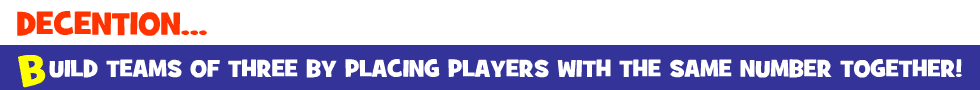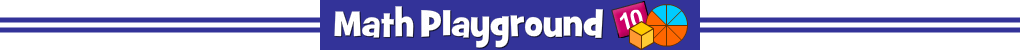Make groups of equal value.
Each group must contain a fraction, a decimal and a percent.
Decention - Learning Connections
Essential Skills
Problem Solving - match fractions, decimals and percents

Common Core Connection for Grades 4 and 5
Use decimal notation for fractions with denominators 10 or 100.
Compare two decimals to hundredths by reasoning about their size.
Compare two fractions with different numerators and different denominators.
More Math Games to Play